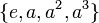# Modular representation theory of cyclic group:Z4

## Contents

View modular representation theory of particular groups | View other specific information about cyclic group:Z4

This article describes the modular representation theory of cyclic group:Z4, i.e., the linear representation theory in characteristic two (this is the only prime wherewe have interesting modular behavior, because it is the only prime dividing the order of the group).

For the linear representation theory in other characteristics, see linear representation theory of cyclic group:Z4.

## Summary

Item Value
degrees of irreducible representations (or equivalently, degrees of irreducible Brauer characters) 1
maximum: 1, lcm: 1, number: 1
smallest field of realization of irreducible representations in characteristic 2 field:F2, i.e., the field of two elements
degrees of indecomposable representations 1,2,3,4
maximum: 4
smallest field of realization of indecomposable representations in characteristic 2 field:F2, i.e., the field of two elements

## Irreducible representations

There is a unique irreducible representation: the trivial representation, which sends all elements of the group to the matrix$( 1 )$. This is a general feature common to all representations of a group of prime power order in a field of characteristic equal to the prime.

## Indecomposable representations

The indecomposable representations are given below. For simplicity, we denote the elements of the group as$\{ e, a, a^2, a^3 \}$ where$e$ is the identity element and$a$ is a generator. Alternatively, we can use the notation of the integers mod 4 and write$0$ for$e$,$1$ for$a$,$2$ for$a^2$,$3$ for$a^3$.

Representation Degree Image of identity element$e$ (or$0$) Image of generator$a$ (or$1$ Image of$a^2$ (or$2$) Image of$a^3$ (or$3$)
trivial 1$(1)$$(1)$$(1)$$(1)$
two-dimensional indecomposable 2$\begin{pmatrix} 1 & 0 \\ 0 & 1 \\\end{pmatrix}$$\begin{pmatrix} 1 & 1 \\ 0 & 1 \\\end{pmatrix}$$\begin{pmatrix} 1 & 0 \\ 0 & 1 \\\end{pmatrix}$$\begin{pmatrix} 1 & 1 \\ 0 & 1 \\\end{pmatrix}$
three-dimensional indecomposable 3$\begin{pmatrix} 1 & 0 & 0 \\ 0 & 1 & 0 \\ 0 & 0 & 1 \\\end{pmatrix}$$\begin{pmatrix} 1 & 1 & 0 \\ 0 & 1 & 1 \\ 0 & 0 & 1 \\\end{pmatrix}$$\begin{pmatrix}1 & 0 & 1 \\ 0 & 1 & 0 \\ 0 & 0 & 1 \\\end{pmatrix}$$\begin{pmatrix} 1 & 1 & 1 \\ 0 & 1 & 1 \\ 0 & 0 & 1 \\\end{pmatrix}$
four-dimensional indecomposable 4$\begin{pmatrix} 1 & 0 & 0 & 0 \\ 0 & 1 & 0 & 0 \\ 0 & 0 & 1 & 0 \\ 0 & 0 & 0 & 1 \\\end{pmatrix}$$\begin{pmatrix} 1 & 1 & 0 & 0 \\ 0 & 1 & 1 & 0 \\ 0 & 0 & 1 & 1 \\ 0 & 0 & 0 & 1 \\\end{pmatrix}$$\begin{pmatrix} 1 & 0 & 1 & 0 \\ 0 & 1 & 0 & 1 \\ 0 & 0 & 1 & 0 \\ 0 & 0 & 0 & 1 \\\end{pmatrix}$$\begin{pmatrix} 1 & 1 & 1 & 0 \\ 0 & 1 & 1 & 1 \\ 0 & 0 & 1 & 1 \\ 0 & 0 & 0 & 1 \\\end{pmatrix}$